# 快速排序算法的简单理解

## 快速排序算法的简单理解

### 递归

``````def countdown(i):
print(i)
countdown(i-1)
``````

``````def countdown(i):
print(i)
if i > 0:	# 递归条件
countdown(i-1)
else:      # 基线条件
return
``````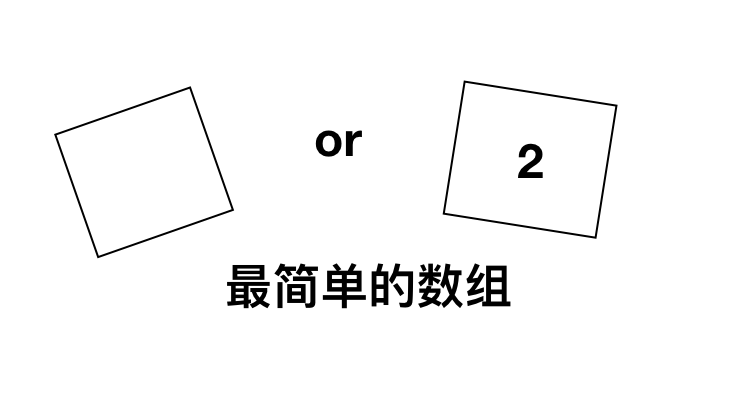只包含一个元素的数组的和，就是它自己，那么两个元素呢？三个元素呢？甚至n个元素呢？ 下面的图解帮助我们更好地理解，输入一个数组，当数组的个数等于1时停止调用，返回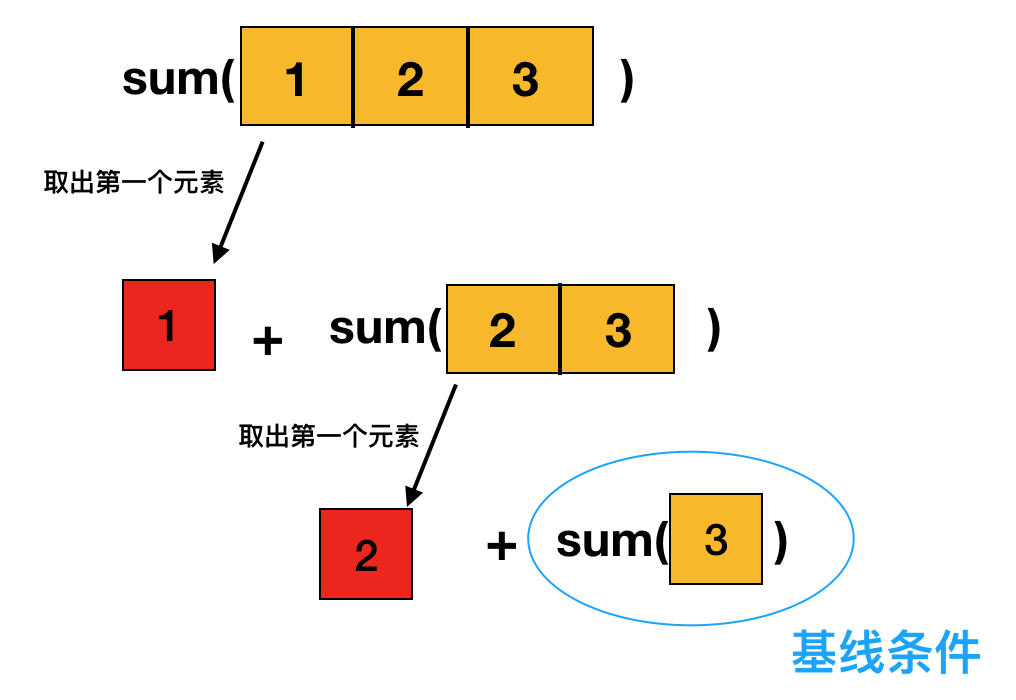``````def sum(arr):
if len(arr) == 1:
return arr
else:
return arr + sum(arr[1:])
``````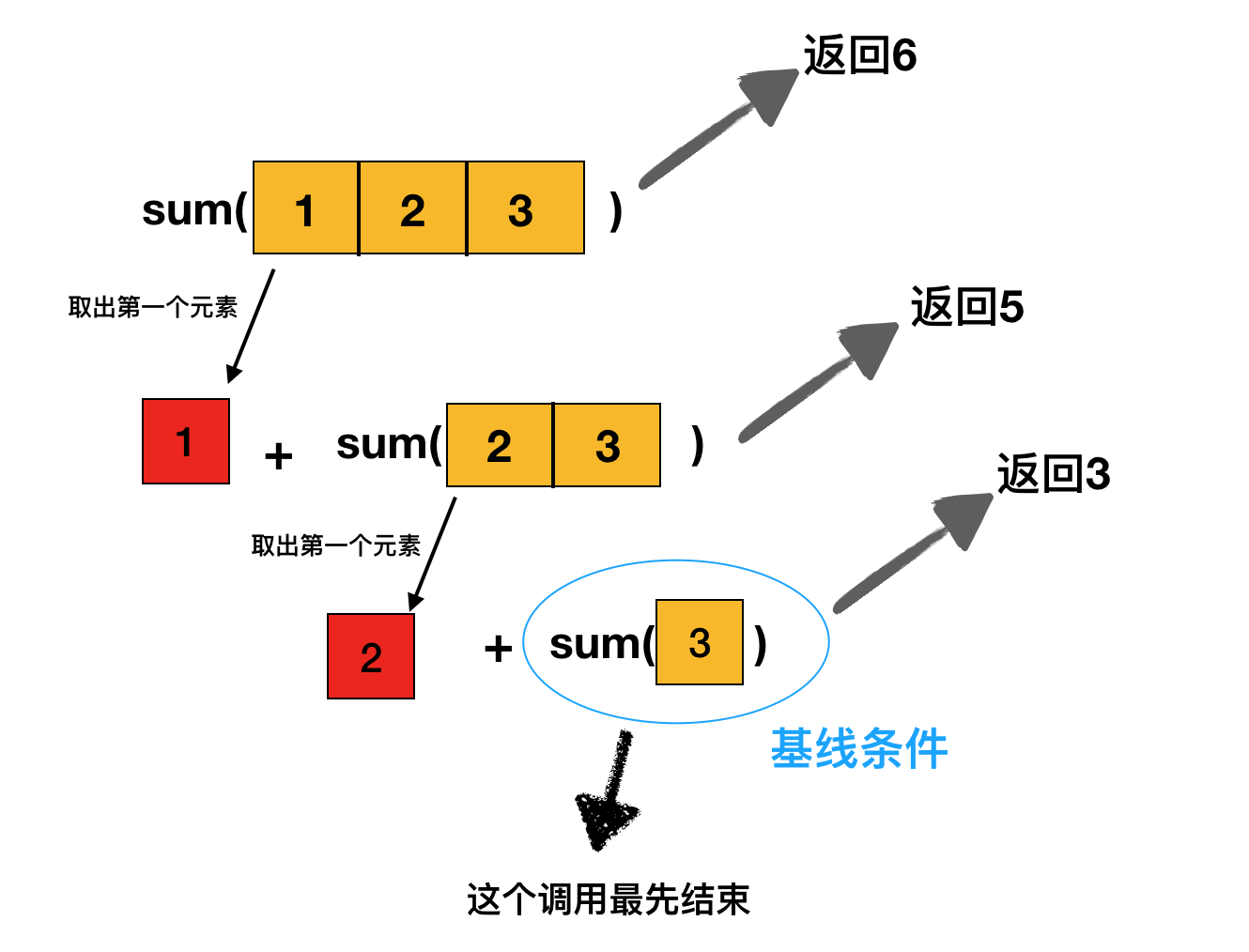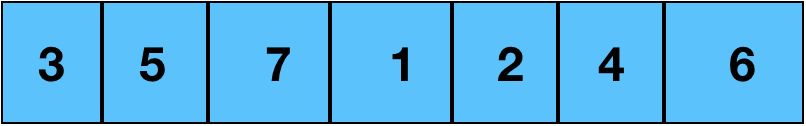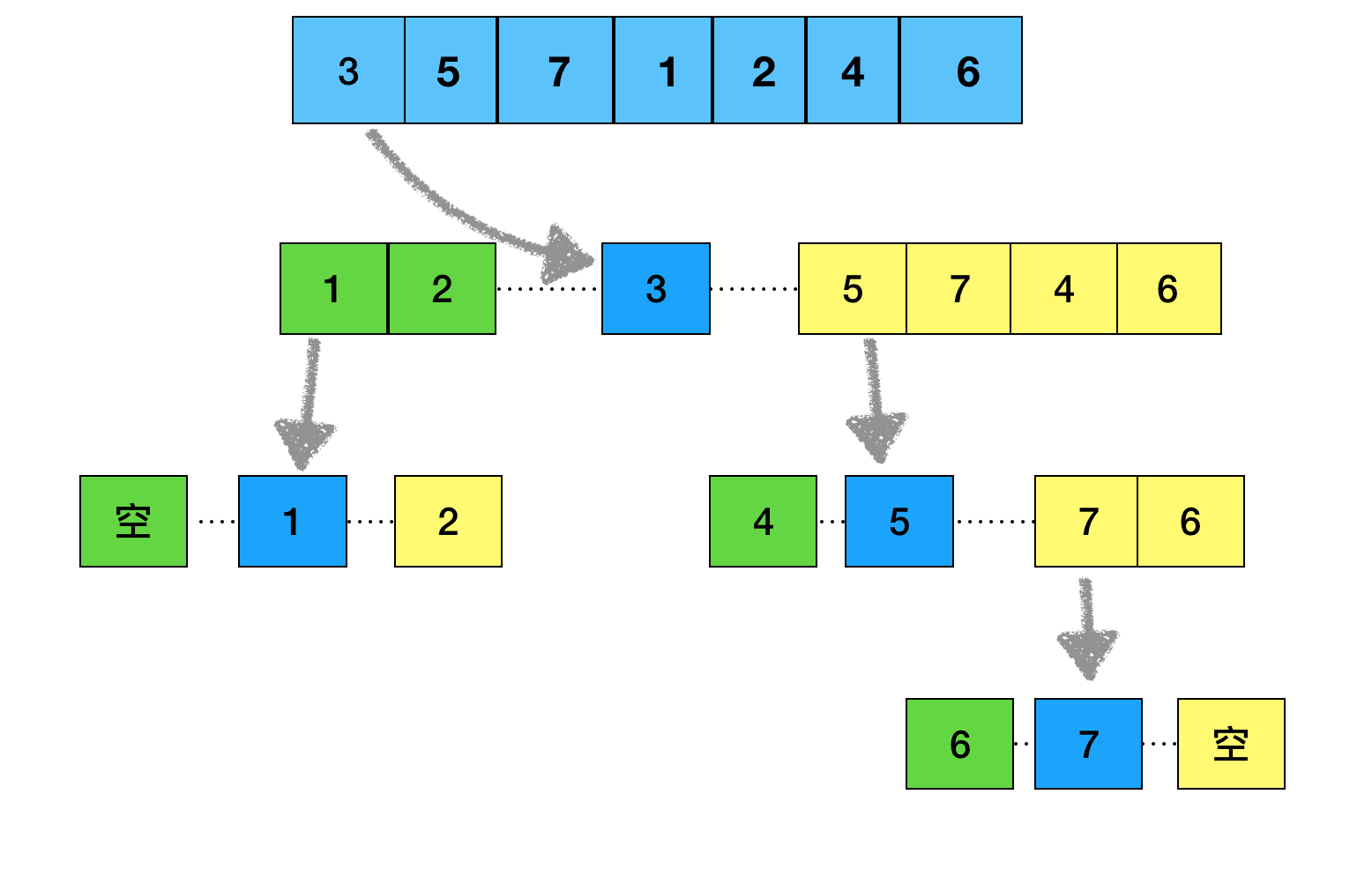``````def quicksort(arr):
if len(arr) < 2:
return arr
else:
min = [x for x in arr[1:] if x < arr]
max = [x for x in arr[1:] if x >= arr]
return quicksort(min) + [arr] + quicksort(max)
``````